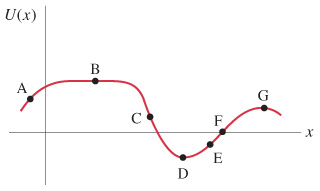# Problem: The figure shows a potential energy curve, U(x). At which point does the force have greatest magnitude?For each labeled point, state whether the force acts to the left or to the right, or is zero.Where is there equilibrium and of what type is it?

⚠️Our tutors found the solution shown to be helpful for the problem you're searching for. We don't have the exact solution yet.

###### Problem Details

The figure shows a potential energy curve, U(x).At which point does the force have greatest magnitude?

For each labeled point, state whether the force acts to the left or to the right, or is zero.

Where is there equilibrium and of what type is it?